# RBSE Solutions For Class 10 Science Chapter 9: Light | Textbook Important Questions & Answers

The RBSE Class 10 Science Chapter 9 solutions are provided here. Students should refer to the solutions when they get doubtful while solving the questions from the textbook. These solutions aim to present a robust and extensive set of solutions for Class 10 Science Chapter 9. Students have been advised to practice these RBSE Class 10 solutions of Chapter 9 to attain good marks in the examinations and reach success. We, at BYJU’S, have solved these solutions with the help of a team of subject matter experts.

These solutions cover all the essential topics and provide explanatory diagrams, according to the question. Chapter 9 – Light covers topics such as reflection of light, refraction of light, concave lens, convex lens, about the human eye, etc. All these topics are compiled in these RBSE solutions for Class 10, so that students can blindly rely on them while preparing for their exam.

### RBSE Class 10 Science Chapter 9: Objective Textbook Questions and Solutions

Q1. Which mirror will show a wide area view?

(A) Plain mirror

(B) Convex mirror

(C) Concave mirror

(D) Parabolic mirror

Q2. Speed of light will be maximum in

(A) Water

(B) Glass

(C) Vacuum

(D) Glycerine

Q3. A coin at the bottom of a water tank appears to be raised because of the phenomenon of

(A) Refraction

(B) Reflection

(C) Total internal reflection

(D) None of these

Q4. The focal length of a mirror is +60 cm then the mirror is a?

(A) Concave mirror

(B) Parabolic mirror

(C) Plain mirror

(D) Convex mirror

Q5. Focal length of a plain mirror is

(A) 0

(B) 1

(C) Infinity

(D) None of these

Q6. Image in a convex mirror will always be

(A) Real and upright

(B) Real and inverted

(C) Virtual and inverted

(D) Virtual and upright

Q7. The power of a lens is +2 Dioptre. What will be its focal length?

(A) 2m

(B) 1m

(C) 0.5m

(D) 0.2m

Q8. In hypermetropia a person

(A) can see nearby object

(B) can see far objects clearly

(C) Neither nearby objects nor far objects are clearly visible

(D) None of these

Q9. To get a real image of the same size of object, where shall the object be placed before a convex lens of focal length 15cm?

(A) 30cm

(B) 15cm

(C) 60cm

(D) None of these

Q10. An object is placed at infinity with respect to a concave lens of focal length 20 cm. Distance of virtual image from lens will be?

(A) 10 cm

(B) 15 cm

(C) 20 cm

(D) At Infinity

### RBSE Class 10 Science Chapter 9: Very Short Answer Type Questions and Solutions

Q11. When an object absorbs all colours of light, then which colour of the object will be seen by us?

Answer: The colour of the object will be black.

Q12. To see our full image in a plane mirror what should be the lowest height of the mirror?

Q13. A light ray is incident on a plane mirror at an angle of 30o. What will be the angle between the reflected and incident ray?

Answer: The angle between the reflected and incident ray will be 60o.

Q14. Write two uses of a convex mirror.

Answer: The two uses of convex mirror are:

• Vehicle mirrors
• Magnifying glass

Q15. Write two uses of a concave mirror.

Answer: The two uses of a concave mirror are:

• Used in shaving mirrors
• Concave mirrors used in headlights

Q16. Write mirror formula?

Answer: Mirror formula – 1/u + 1/v = 1/f

Q17. Write the relation between radius of curvature and focal length of a spherical mirror.

Answer: f = R/2 where f is the focal length and R is the radius of curvature of the spherical mirror.

Q18. Give the magnification formula

Answer: m = hi/ho = v/u

Where, m= magnification

hi = height of image

ho= height of object

Q19. Give snell’s law.

Answer: Snell’s law is defined as “The ratio of the sine of the angle of incidence to the sine of the angle of refraction is a constant, for the light of a given colour and for the given pair of media”. Snell’s law formula is expressed as: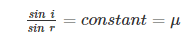Q20. Write lens formula.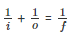where,

i= distance of image from the lens

o= distance of object from the lens

f= focal length of the lens

Q21. Parallel rays from an object are incident on a convex lens. Where will the image be formed?

Answer: The image will be formed at focus.

Q22. What is the unit of power of lens?

Answer: Dioptre is the SI unit for measuring power of a lens.

Q23. In which conditions a person with myopia cannot clearly see the object?

Answer: A person with myopia cannot clearly see distant objects.

Q24. Which defect of eye can be rectified with the help of a convex lens of appropriate power.

Answer: Long sightedness of hypermetropia can be rectified with the help of a convex lens of appropriate power.

Q25. What is cataract?

Answer: Cataract is a defect which occurs due to formation of an opaque or cloudy membrane over eye lens which can be cured only by surgery.

Q26. What will be the nature of our image in a shaving mirror?

Answer: It will be virtual, enlarged and erect.

### RBSE Class 10 Science Chapter 9: Short Answer Type Questions and Solutions

Q27. What do you mean by regular reflection and diffused reflection?

Answer: Specular Reflection or regular reflection refers to a clear and sharp reflection, like the ones you get in a mirror. A mirror is made of glass which is coated with a uniform layer of a highly reflective material such as powder. This reflective surface reflects almost all the light incident on it uniformly. There is not much variation in the angles of reflections between various points. This means that the haziness and the blurring are almost entirely eliminated.

Reflective surface other than mirrors, in general, has a very rough finish. This may be due to wear and tear such as scratches and dents or dirt on the surface. Sometimes even the material of which the surface is made of matters. All this leads to a loss of both the brightness and the quality of the reflection.

In case of such rough surfaces, the angle of reflection when compared between points is completely haphazard. For rough surfaces, the rays incident at slightly different points on the surface is reflected in completely different directions. This type of reflection is called diffused reflection and is what enables us to see non-shiny objects.

Q28. Explain lateral inversion?

Answer: The phenomenon where your left appears as the right and vice versa in a mirror is referred to as Lateral Inversion. The lateral inversion we experience is not caused by the mirror but by our perception of the mirror image. What causes the lateral inversion is how we see it. Your brain when it sees a mirror image tries to make sense of it. In doing so, it imagines that another you has walked to the mirror and is standing in front of you from behind the mirror. This means that contrary to how we see mirror images, a mirror does not reverse left and right. Instead, it reverses the front and back. Where your back should be your face is and your brain assumes this to be another person standing in front of you and assumes the left and right reversal i.e. lateral inversion.

Q29. An object is placed between radius of curvature and focus of a concave lens, show the image formation by ray diagram.

Answer: When an object is placed in between the center of curvature and focus, the real image is formed behind the center of curvature. The size of the image is smaller as compared to that of the object.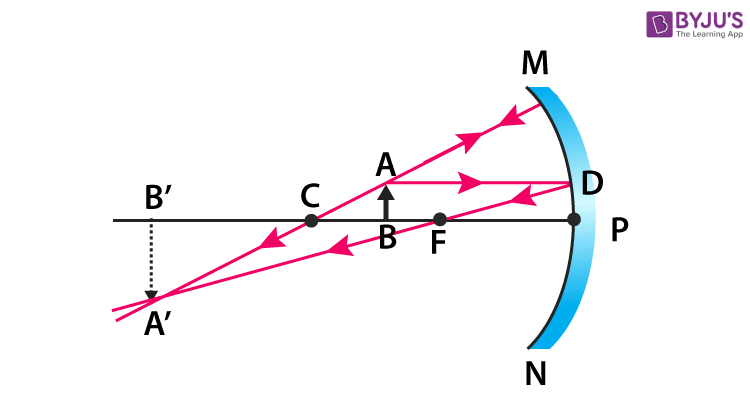Q30. Explain Cartesian sign convention for spherical mirrors?

Answer: The Cartesian sign convention for spherical mirrors are as follows

• All distances are measured from the optical centre of the lens.
• Distances measured in the direction opposite to the direction of incident light are considered negative.
• Distances measured in the same direction as the incident light are considered positive.
• Heights measured upwards and perpendicular to the principal axis are considered positive.
• Heights measured downwards and perpendicular to the principal axis are considered negative.

Q31. Discuss refraction of light and write the laws of refraction.

Answer: Refraction is the bending of a wave when it enters a medium where its speed is different. It is the change in direction of a wave passing from one medium to another or from a gradual change in the medium. Refraction of light is one of the most usually observed phenomena which includes refraction of light through prism, but other waves like sound waves and water waves also experience refraction.

Laws of refraction state that:

• The incident ray, refracted ray, and the normal to the interface of two media at the point of incidence all lie on the same plane.
• The ratio of sine of angle of incidence to the sine of angle of refraction is a constant. This is also known as Snell’s law of refraction.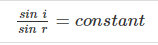Q32. What are the different types of concave lens and convex lens?

Answer: The different types of concave lens and convex lens are:

Concave lens – Biconcave lens, plano-concave lens, convexo-concave lens

Convex lens – Biconvex lens, piano-convex lens, concavo-convex lens

Q33. Define principal focus and optical centre of a spherical lens.

Answer: A number of rays which falls parallel to the principal axis after getting reflected, the rays seems to come from a point on the principal axis. This is called Principal focus Convex Mirror. A number of rays which falls parallel to the principal axis on a concave mirror meets at a point on the principal axis after getting reflected. This is known as the principal focus of a concave mirror.

Optical Centre is a point within the lens where the diameter of the lens and the principal axis meet.

Q34. What do you mean by radius of curvature and centre of curvature of a spherical lens.

Answer: Centre of Curvature – The point in the centre of the mirror that passes through the curve of the mirror and has the same tangent and curvature at that point.

Radius of curvature: It’s the linear distance between Pole and the Center of curvature.

Q35. Write the laws of refraction for spherical mirrors?

Answer: The laws of refraction for spherical mirrors are as follows:

• Incident rays parallel to principal axis of a concave lens converge at focus after refraction. When such parallel rays are incident on a concave lens then they diverge and when extended backwards they meet at focus or we can say that they seem to emerge from the focal point
• When light rays pass through focus before their incidence on curved surface of a convex lens then such rays become parallel to principal axis after refraction. When light rays are incident on a concave lens in such direction that they seem like they are going towards focus then after refraction such rays become parallel to principal axis.
• When light rays pass through optical centre of a lens then the rays remain undeviated after refraction.

Q36. Explain the image formation by a concave lens with ray diagram.

Answer: The image formation by a concave lens with ray diagram are as follows:

When an object is placed at infinity, a virtual image is formed at the focus. The size of the image is much smaller as compared to that of the object.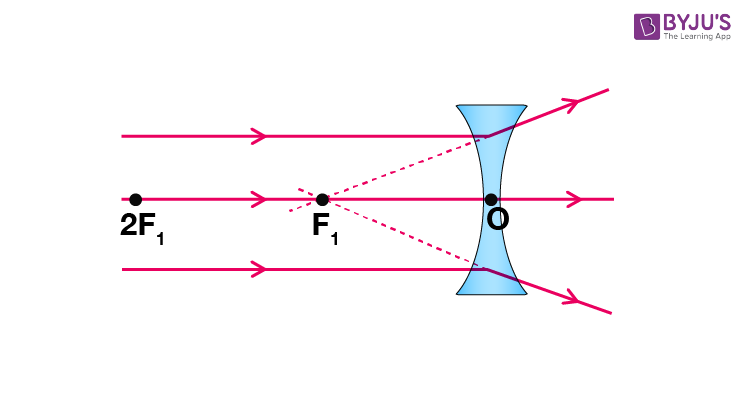When an object is placed at a finite distance from the lens, a virtual image is formed between pole and focus of the convex lens. The size of the image is smaller as compared to that of the object.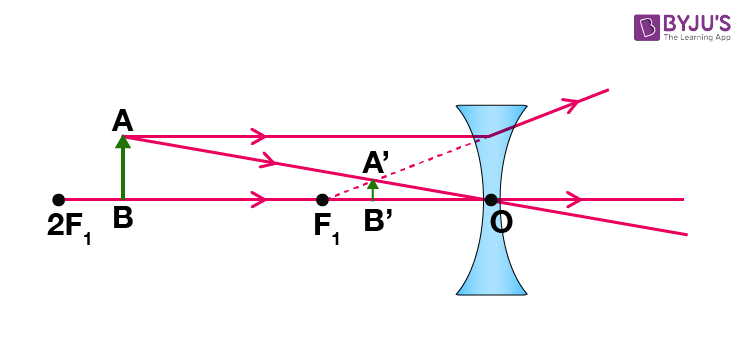Q37. What do you mean by the power of a lens?

Answer: Power of a Lens is one of the most interesting concepts in ray optics. The detailed concept of this topic is given in the below article so that learners can understand this chapter more effectively.

Simply put, the power of a lens in Ray Optics is its ability to bend light. The greater the power of a lens, the greater is its ability to refract light that passes through it. For a convex lens, the converging ability is defined by power and in a concave lens, the diverging ability.

Q38. What do you mean by myopia? How is it corrected?

Answer: Myopia or Nearsightedness occurs when the eye loses its ability to focus on far-off objects as the lenses do not possess a long focal length. Objects that are near are clearly visible to patients of this defect. As we know, from the reference for countless ray diagrams describing the functioning of the eye, when the light suffers higher refraction than usual, the eye would not be able to form an image for far away objects.

A diverging lens can be used to fix the problem of nearsightedness.

Q39. What is hypermetropia? How is this defect corrected?

Answer: Farsightedness is also known as hyperopia. It is a refractive error in which distant objects are clearly seen, but close objects appear blurry. People experience farsightedness differently, and some people, more commonly when they are young, do not notice any problems with their vision. In addition, for people with significant farsightedness, vision can be blurry for objects at any distance, near or far.

This defect can be corrected by using a convex lens of suitable focal length.

Q40. What do you mean by presbyopia and astigmatism?

Answer: Under normal circumstances, when light enters a person’s eye which is naturally shaped like a sphere it creates a clear view of the object as the light is refracted evenly. However, if the eye of a person is curved but not completely spherical, like the back of a spoon, what happens? For this person, when light enters the eye it is refracted more in one direction than the other, allowing only part of the object to be in focus at one time. This is called a refractive error. Objects at any distance can appear blurry and wavy.

This defect in the eye or in a lens caused by a deviation from spherical curvature, which results in distorted images, as light rays are prevented from meeting at a common focus is called Astigmatism.

Presbyopia is caused as part of the natural aging process of the eye. The ciliary muscles lose their ideal functioning power over time and do not work effectively. Even the crystalline lens loses its flexibility over time (it starts hardening), thereby affecting its focusing power. Even if someone has never had any vision problems in their life, they may still very well be subject to the effects of presbyopia. It occurs between the ages of 40 – 60.

Q41: What is the power of accommodation of an eye and what is the range of the vision?

Answer: The process by which certain muscles (called ciliary muscles) function, to change the focal length of the eyes so that the image is clearly formed on the retina is called the accommodation of the eye. This will vary for near and distant objects and also for objects moving away or towards the eye. By adjusting the focal length, the eye is actually changing its lens power. This is called the accommodating power of the eye.

The Power of accommodation for a person with normal eyesight is around 4 dioptre (unit of lens power).

Q42. An object is placed on principal axis between infinity and 2F1 of a convex lens. Show the image formation with ray diagram.

Answer: When object is at finite distance shows position of image for different positions of object.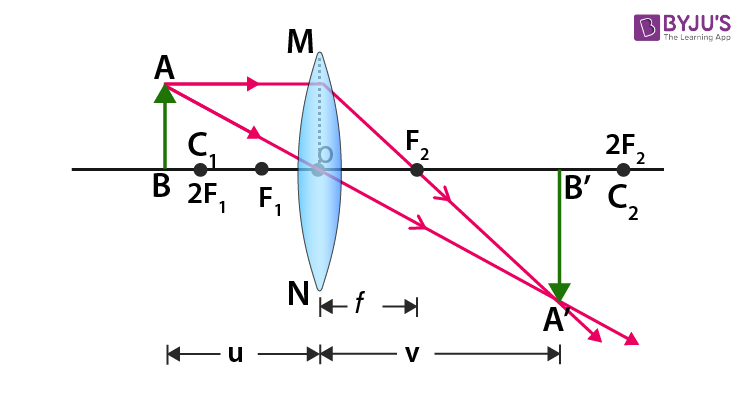### RBSE Class 10 Science Chapter 9: Essay Type Questions and Solutions

Q43. With the help of ray diagram, explain the position and nature of image for following positions of object for a concave mirror.

(i) When the object is between infinity and centre of curvature.

(ii) When the object is at the centre of curvature

(iii) When the object is between centre of curvature and focus

(iv) When the object is at focus.

(v) When the object is between focus and pole.

 S.No Position of Object Position of Image Size of Image Nature of Image 1 At infinity At the focus F Highly Diminished Real and Inverted 2 Beyond the centre of curvature C Between F and C Diminished Real and Inverted 3 At the centre of curvature C At C Same Size Real and Inverted 4 Between C and F Beyond C Enlarged Real and Inverted 5 At focus F At Infinity Highly Enlarged Real and Inverted

Concave Mirror Ray Diagram

When an object is placed at infinity, a real image is formed at the focus. The size of the image is much smaller as compared to that of the object.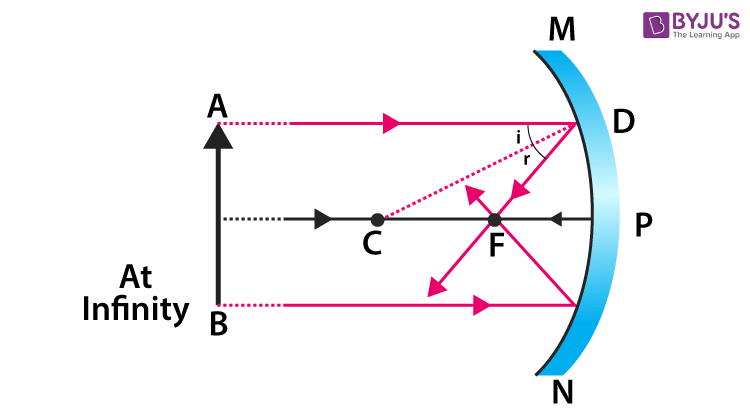When an object is placed behind the center of curvature, a real image is formed between the center of curvature and focus. The size of the image is smaller as compared to that of the object.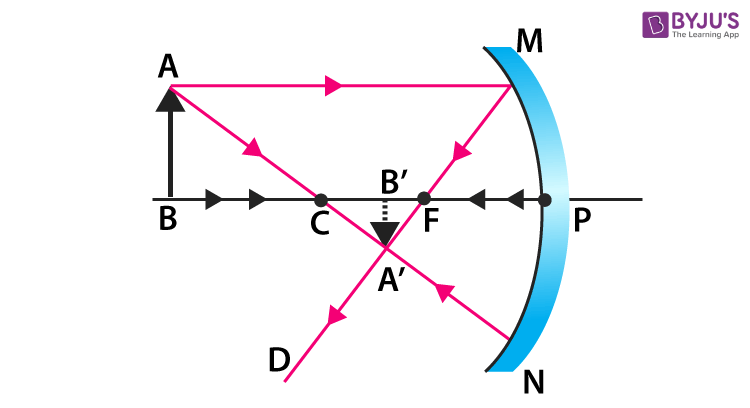When an object is placed at the center of curvature and focus, the real image is formed at the center of curvature. The size of the image is same as compared to that of the object.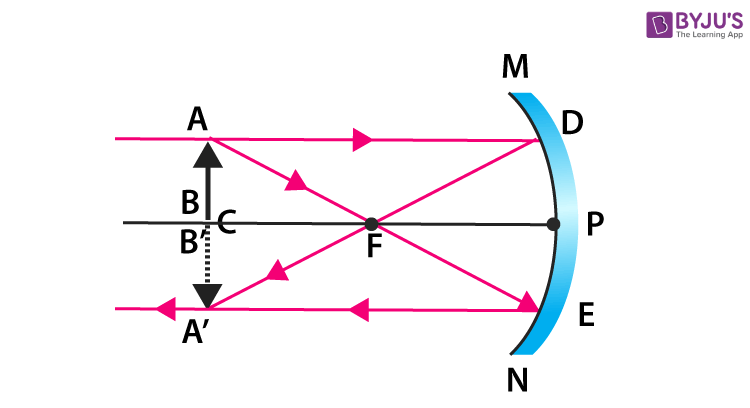When an object is placed in between the center of curvature and focus, the real image is formed behind the center of curvature. The size of the image is smaller as compared to that of the object.When an object is placed at the focus, the real image is formed at infinity. The size of the image is much larger as compared to that of the object.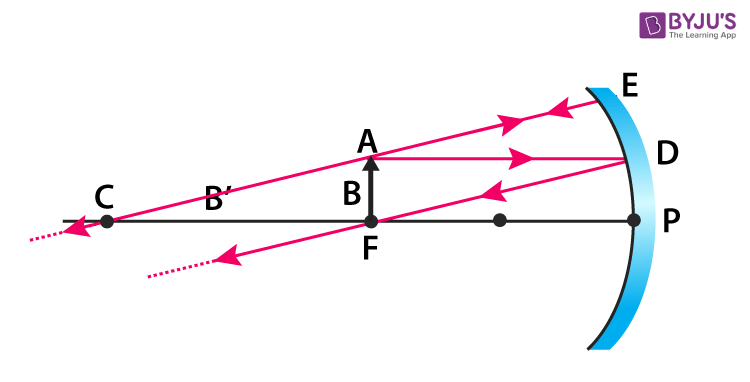When an object is placed in between focus and pole, a virtual image is formed. The size of the image is larger as compared to that of the object.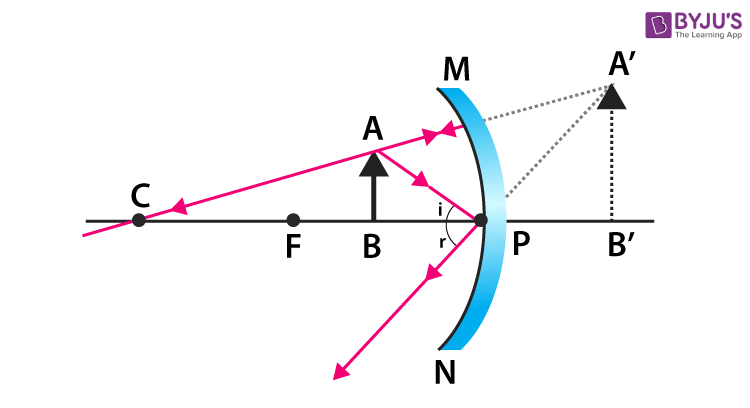Q44. What do you mean by refraction? Write laws of refraction and explain the refraction of light rays with the help of a glass slab.

Answer: Refraction of Light is a phenomenon wherein light bends and travels from one transparent substance to another. We can define refraction as the phenomenon of bending of light when it passes from one substance to another. Rainbow, mirage are a few real-life examples of refraction. Sunrise and sunset is a result of atmospheric refraction. Let us understand it more clearly by the concept of refraction through a rectangular glass slab.

Refraction through a rectangular glass slab:

Let us understand the phenomenon of refraction of light with the help of an activity.

1. Take a white sheet and put a glass slab over the sheet.
2. Draw the outline of the slab and mark it as ABCD as shown in the figure.
3. Take two pins, say E and F and put it at the edge of A and B.
4. Now, look at the images of the pins E and F through the opposite sides. Place two more pins G and H such that G, F and the images of E and F are in the same straight line.
5. Remove the pins and the slab.
6. Join the points E and F and extend the line up to AB. EF meets at O. In the same manner, join G and H and extend it to the edge of CD. Mark O’ the point where HG meets CD.
7. Now, join O and O’. Also, extend EF till P as shown in the figure.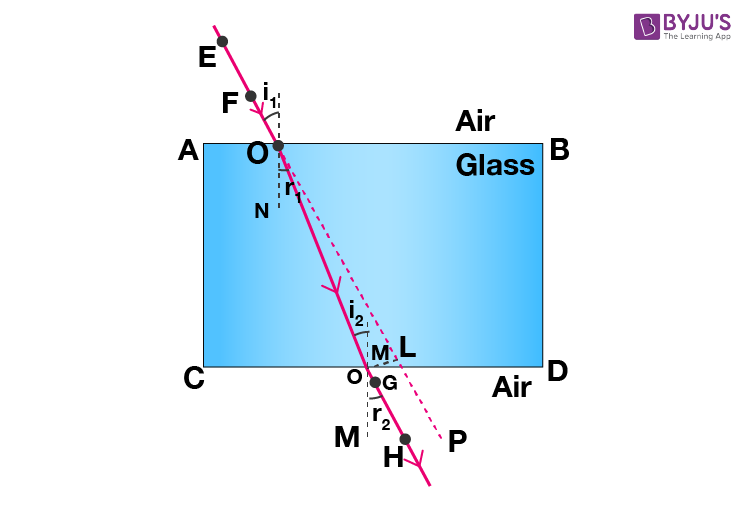In the above activity, we have observed that light rays change their direction at O and O’ points at the surfaces of two separating transparent media. Make a perpendicular line NN’ to AB at O and another perpendicular line MM’ to CD at O’. At O the light ray enters from a rarer medium to a denser medium i.e. from air to glass. Hence the light travels towards the normal. While at O’, the ray of light moves from denser to rarer medium. Hence the light moves away from the normal.

In the given figure EO is the incident ray, O’H is the emergent ray and the OO’ is the refracted ray. In this, we can observe that emergent ray is parallel to the incident ray. From the above activity, we can say that refraction happens due to change in the speed of light when light travels from one medium to another.

Laws of refraction

The laws of refraction govern the behaviour of light as they pass through the interface between two media. It is generally known as Snell’s Law. From the above-depicted activity, we can say that refraction of light follows two laws:

• The refracted ray, incident ray and the normal at the interface of two transparent media at the point of incidence, all lie in the same plane.
• For the given pair of media, the ratio of the sine of the angle of incidence to the sine of angle refraction is always constant.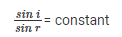Q45. With the help of ray diagram explain the nature and position of the image for the following positions of object for convex mirror.

(i) When an object is at infinity.

(ii) When the object is at a certain distance.

Answer (i): When an object is placed at infinity, a virtual image is formed at the focus. The size of the image is much smaller as compared to that of the object.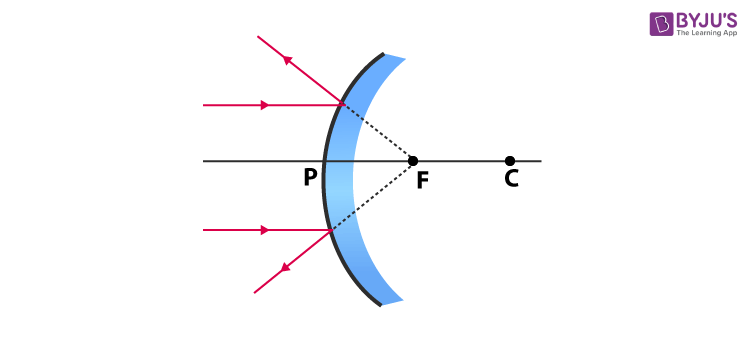Answer ii: When an object is placed at a finite distance from the mirror, a virtual image is formed between pole and focus of the convex mirror. The size of the image is smaller as compared to that of the object.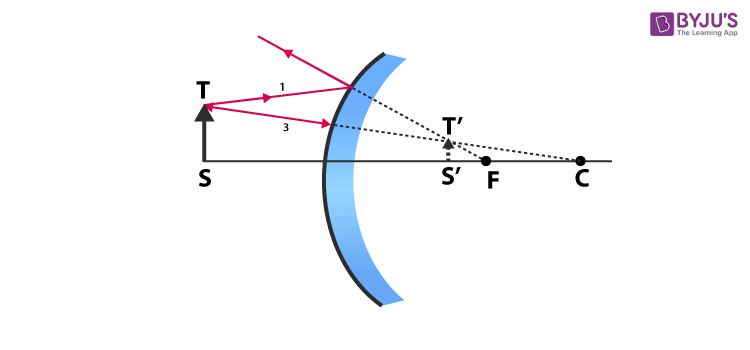Q46. Explain the nature and position of image with the help of ray diagram for a concave lens when object is placed.

(i) at focus point of lens

(ii) between focus F1 and 2F1

(iii) Between 2F1 and infinity.

Answer (i): Image formed at infinity, highly enlarged, real and inverted.

Answer (ii): Image is formed beyond 2F2 . Image is enlarged, real and inverted

Answer (iii): Image is formed between F2 and 2F2 . Image is smaller, real and inverted.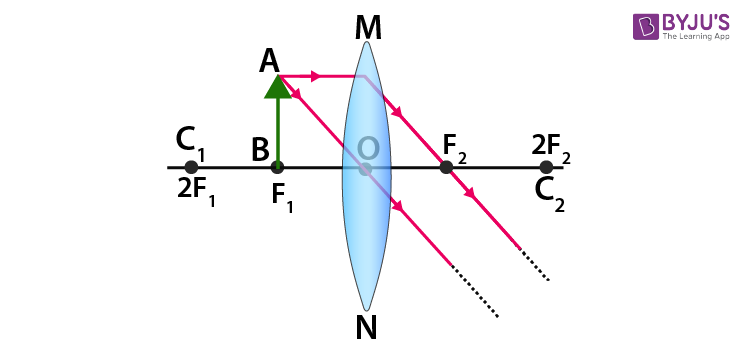Q47. For a convex lens explain the formation of image and nature of image with ray diagram when object is placed.

(i) between focus and optical centre

(ii) at focus F1

(iii) between F1 and 2F1

(iv) at 2F1

Answer (i): When an object is placed in between focus and pole, a virtual image is formed. The size of the image is larger as compared to that of the object.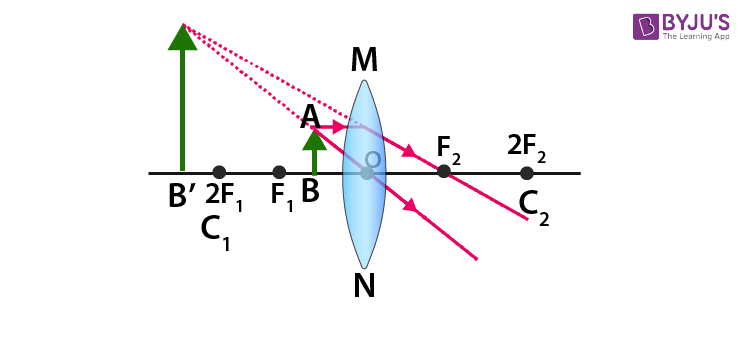Answer (ii): When an object is placed at the focus, a real image is formed at infinity. The size of the image is much larger as compared to that of the object.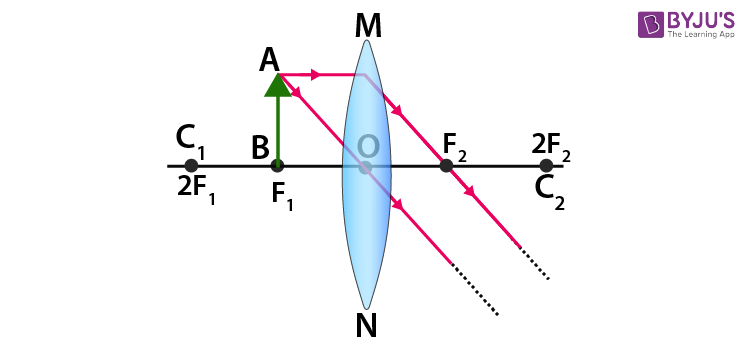Answer (iii): When an object is placed in between the center of curvature and focus, the real image is formed behind the center of curvature. The size of the image is smaller as compared to that of the object.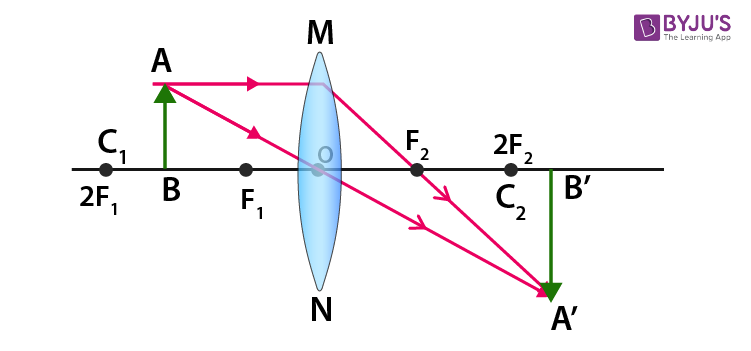Answer iv: When an object is at the center of curvature, the real image is formed at the other center of curvature. The size of the image is same as compared to that of the object.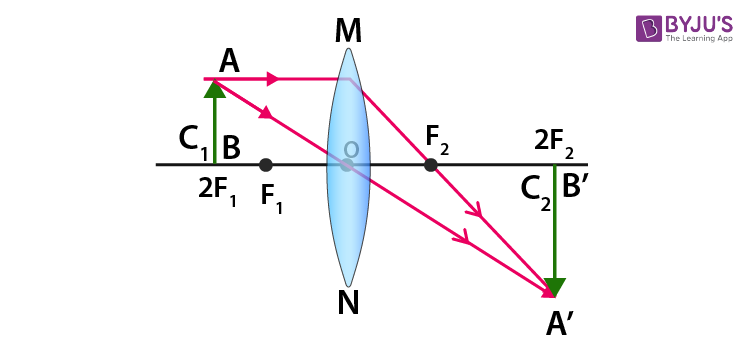Q48. Discuss in detail in different types of defects in vision and methods to correct the defects of vision.

Answer: The different types of defects in vision are as follows:

• Myopia
• Hypermetropia
• Presbyopia
• Astigmatism

Myopia – Myopia or Nearsightedness occurs when the eye loses its ability to focus on far-off objects as the lenses do not possess a long focal length. Objects that are near are clearly visible to patients of this defect. As we know, from the reference for countless ray diagrams describing the functioning of the eye, when the light suffers higher refraction than usual the eye would not be able to form an image for far away objects.

A diverging lens can be used to fix the problem of nearsightedness.

Hypermetropia – People with hyperopia or hypermetropia are said to have farsightedness or longsightedness. In hyperopia image formation of nearby objects appears out of focus but objects far away can be seen clearly.

• By using corrective eyeglasses: Depending on by how much the focal length has been altered due to the effects of hyperopia, powered convex lenses can correct this problem. It is also the safest option with a minor inconveniences
• By using contact lenses: Using this has the same effect as that of using eyeglasses but presents a more convenient and comfortable option. Using contact lenses isn’t safe for everyone, however.
• Refractive surgery: Since the refractive power of the eye is affected by hyperopia, altering this through surgery is another way to counter the defects. An example of refractive surgery is Lasik surgery, which is quite common.

Presbyopia – Presbyopia is caused as part of the natural aging process of the eye. The ciliary muscles lose their ideal functioning power over time and do not work effectively. Even the crystalline lens loses its flexibility over time (it starts hardening), thereby affecting its focusing power. Even if someone has never had any vision problems in their life, they may still very well be subject to the effects of presbyopia. It occurs between the ages of 40 – 60.

Just as in hyperopia, presbyopia can be treated with the use of simple eyeglasses with convex lenses (positive power). Contact lenses can also be used in such a situation. Although they are more convenient, sometimes cases of both myopia and presbyopia may exist. In such a case, it is better to use bi-focal lenses. Bifocals have both a concave and convex surface as part of their refractive design.

Astigmatism – Under normal circumstances, when light enters a person’s eye which is naturally shaped like a sphere it creates a clear view of the object as the light is refracted evenly. However, if the eye of a person is curved but not completely spherical, like the back of a spoon, what happens? For this person, when light enters the eye it is refracted more in one direction than the other, allowing only part of the object to be in focus at one time. This is called a refractive error. Objects at any distance can appear blurry and wavy.

This defect in the eye or in a lens caused by a deviation from spherical curvature, which results in distorted images, as light rays are prevented from meeting at a common focus is called Astigmatism.

### RBSE Class 10 Science Chapter 9: Numerical Questions and Solutions

Q49. Focal length of a concave mirror is 30cm. If an object is placed at 40cm then find the position of image and also get the magnification (-120cm, 3times & real).

u = -40cm

So, by using the formula image distance can be calculated as

1/v + 1/u = 1/f

1/v – 1/40 = -1/30

1/v = -1/30 + 1/40

= – 4 + 2/ 120

= – 1/120

So, v = -120cm

Magnification can be calculated by using the formula

M = v/u

= -120/-40

= 3

The answer with a positive sign signifies that the image is real.

Q50. An image of an object is seen at 8cm from the convex mirror. If focal length of convex mirror is 16cm then find the position of the object (-16cm).

f = 16cm

We can calculate object distance by using the formula

1/v + 1/f = 1/f

1/8 + 1/u = 1/16

1/u = 1/16 – 1/8

= 1 – 2/16

= -1/16

u = -16cm

Q51. An object is placed at a distance of 60cm from the convex lens of focal length 30cm. If object height is 3cm then find the position and nature of the image (60cm, 3cm real and inverted)

f = 30cm

h = 3cm

We can calculate the image distance by

1/v – 1/u = 1/f

1/v + 1/60 = 1/30

1/v = 1/30 – 1/60

= 2 – 1/60 = 1/60

v = 60cm

Now, image height can be calculated by

M = h1/h = v/u

M = h1/3 = 60/-60

h1 = -3cm

Therefore, the result shows that image is of the same size. Negative sign shows that image is real and inverted.

Q52. An object is placed at 10cm from a convex lens. If focal length of convex lens is 40cm then find the position and nature of the image. (-13.33cm, 1.33 times, virtual & upright)

u = -10cm

f = 40cm

Image distance can be calculated as

1/v – 1/u = 1/f

1/v + 1/10 = 1/40

1/v = 1/40 – 1/10

= 1 – 4/40

= -3/40

v = -13.33cm

Magnification = v/u

= 40/ 3 × 10

= 4/3

= 1.33

The results show that the image is enlarged. Positive sign means the image is virtual and erect.

Q53. Focal length of a concave mirror is 30cm. If an object is placed at 20cm from the mirror then find the image position and its nature. (+60cm, 3times and virtual).

f = -30cm

u = -20cm

We can calculate image distance by

1/v + 1/u = 1/f

1/v – 1/20 = -1/30

1/v = -1/30 + 1/20

= -2 + 3/60 = 1/60

v = 60cm

Magnification can be calculated as,

M = -v/u = -60/-20

= 3

Q54. Image of an object placed in front of a concave lens is at 10cm. If focal length of concave lens is 15cm then find the distance object of object from the lens. (-30cm).

v = -10cm, f = -15cm

Object distance can be calculated as,

1/v – 1/u = 1/f

-1/10 – 1/u = -1/15

1/u = -1/10 + 1/15

= -3 + 2/30

= -1/30

u = -30cm

Q55. Find the magnification of a convex lens of focal length 10cm when the upright image of the lens is formed at distance of near point of eye. (3.5)

f = 10cm

v = -25cm

Object distance can be calculated as

1/v – 1/u = 1/f

-1/25 – 1/u = 1/10

1/u = -1/25 – 1/10

= -2 – 5/50

= -7/50

= -50/7

Magnification = v/u = -25 × 7/-50 = 7/2 = 3.5